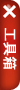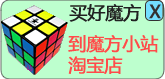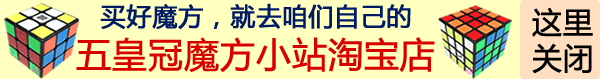SQ-1魔方教程 第2步 调整棱块朝向,角块顺序,棱块顺序和奇偶校验

 SQ-1魔方公式表 调整棱朝向, 交换顶层右面棱和底层后面棱的颜色 (1,0)/(3,0)/(3,0)/(-1,-1)/(-2,1)/(-3,0) 找得到两角同色的边，把两角同色边放在底层的后面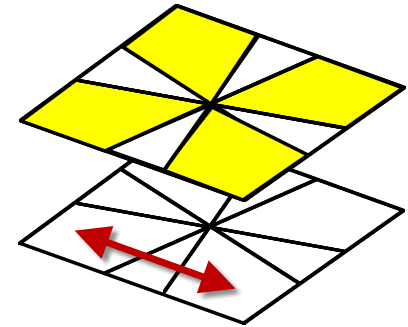/(3,-3)/(0,3)/(-3,0)/(3,0)/(-3,0) 找不到两角同色的边，用这个公式，顶层随便怎么摆都可。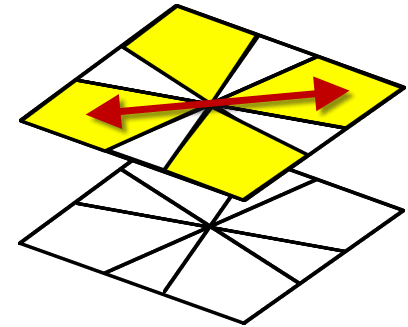/(3,3)/(3,0) 做三次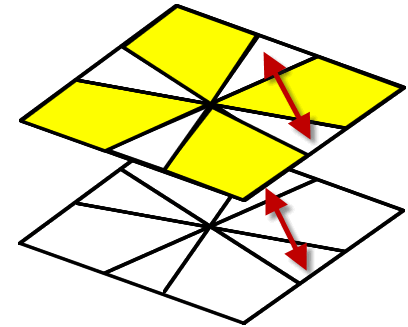(-2,0)/(3,0)/(-1,-1)/(-2,1)/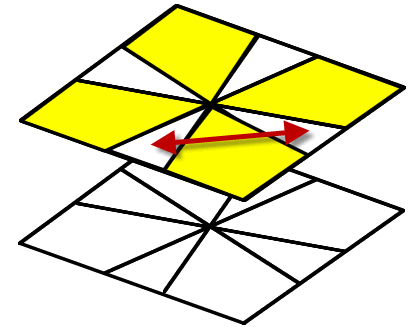奇偶校验公式 /(-3,0)/(0,3)/(0,-3)/(0,3) /(2,0)/(0,2)/(-2,0)/(4,0)/(0,-2)/(0,2) /(-1,4)/(0,-3)/(0,3)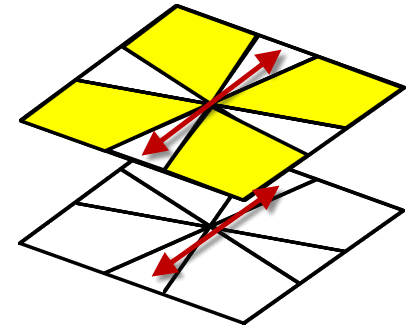M2=(1,0)/(-1,-1)/(0,1); U2=(6,0) 此公式为M2 U2 M2 U2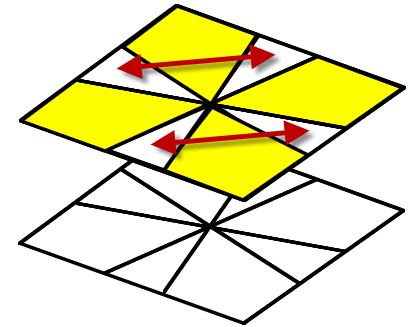M2=(1,0)/(-1,-1)/(0,1); U=(3,0); U'=(-3,0) 此公式为M2 U M2 U' M2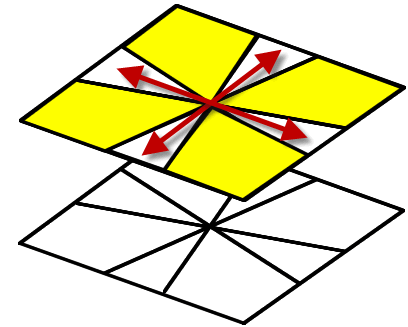M2=(1,0)/(-1,-1)/(0,1); U=(3,0); U2=(6,0) 此公式为M2 U M2 U2 M2 U M2 顶面底面互换 /(6,6)/ 中间层变正方形 /(6,0) 做三次

#### 您可以直接写信给我，任何的只言片语的建议感想都可以的：）我会尽量回复大家。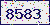验证码看不清楚?请点击验证码图片获得一个新的。